# Problem Solving and Reasoning Lesson 5 Problem Solving

• Slides: 21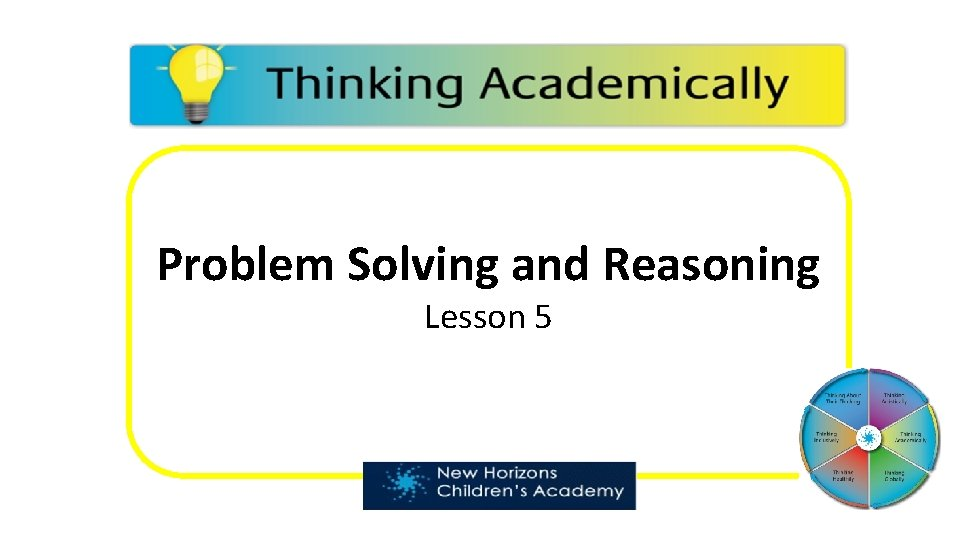Problem Solving and Reasoning Lesson 5Problem Solving and Reasoning Success Criteria ü I can apply my knowledge of shape to a range of questions. ü I can read the questions carefully, identifying the key information. ü I can communicate with clarity and precision, fully explaining my answer when necessary. DNA What if all shapes were irregular?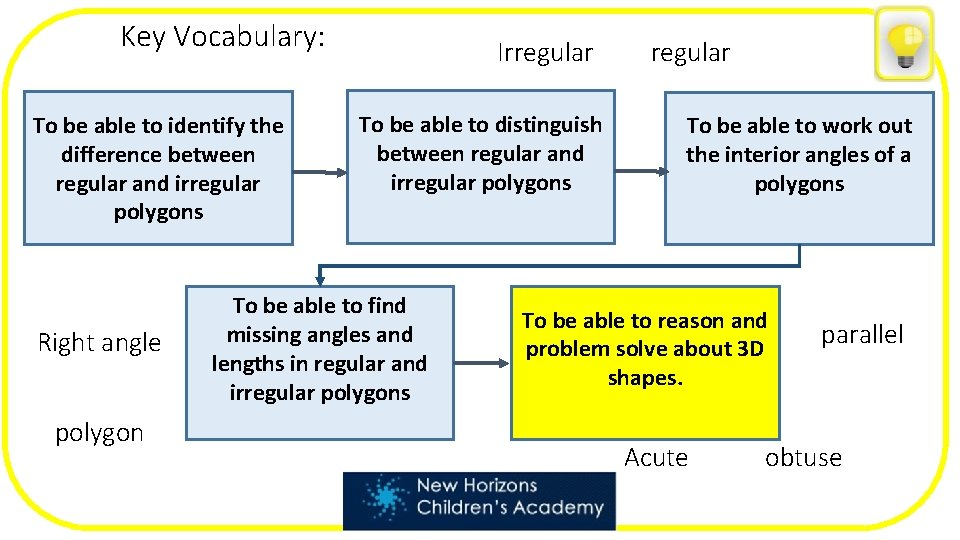Key Vocabulary: To be able to identify the difference between regular and irregular polygons Right angle polygon Irregular To be able to distinguish between regular and irregular polygons To be able to find missing angles and lengths in regular and irregular polygons regular To be able to work out the interior angles of a polygons To be able to reason and problem solve about 3 D shapes. Acute parallel obtuse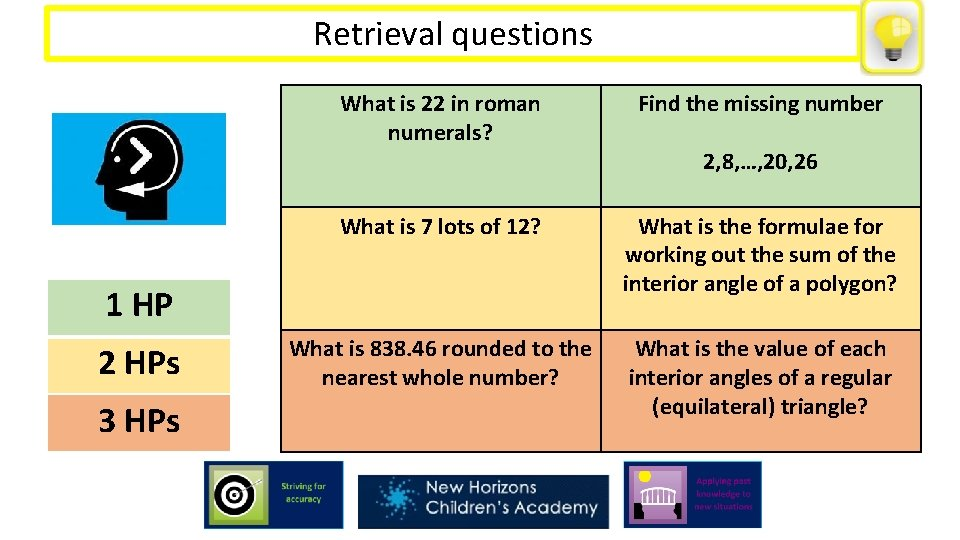Retrieval questions Retrieval practice What is 22 in roman numerals? Find the missing number What is 7 lots of 12? What is the formulae for working out the sum of the interior angle of a polygon? What is 838. 46 rounded to the nearest whole number? What is the value of each interior angles of a regular (equilateral) triangle? 1 HP 2 HPs 3 HPs 2, 8, …, 20, 26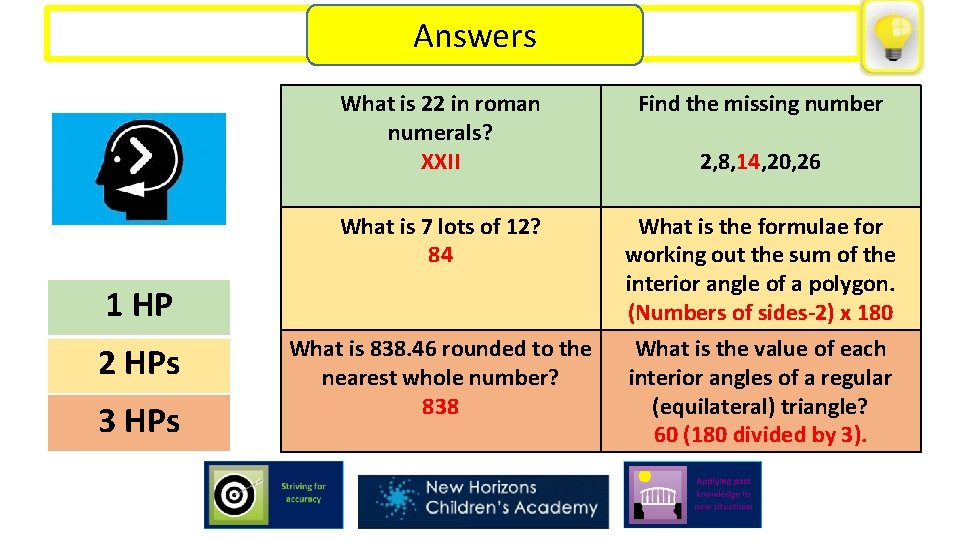Retrieval questions Retrieval practice Answers What is 22 in roman numerals? XXII Find the missing number What is 7 lots of 12? 84 What is the formulae for working out the sum of the interior angle of a polygon. (Numbers of sides-2) x 180 What is the value of each interior angles of a regular (equilateral) triangle? 60 (180 divided by 3). 1 HP 2 HPs 3 HPs What is 838. 46 rounded to the nearest whole number? 838 2, 8, 14, 20, 26Key Words Recap Polygon - A polygon is a 2 D shape with straight sides. For example, a square, a triangle and a hexagon. Regular polygon - A regular polygon is a shape that has equal sides in length and equal angles. Irregular polygon - An irregular polygon is a 2 D shape that does not have equal sides in length and the angles are not equal. © The Digital Stationer 2018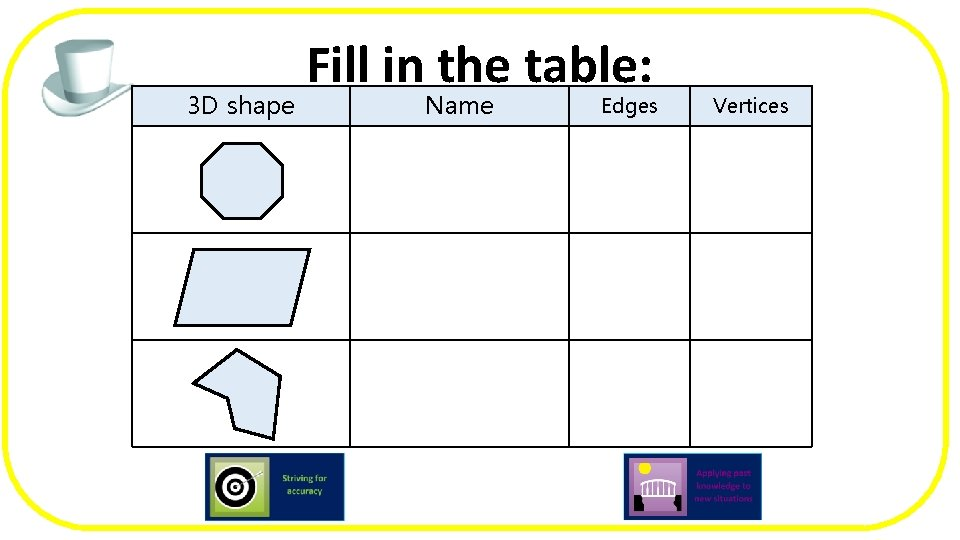3 D shape Fill in the table: Name Edges Vertices3 D shape Fill Answers in the table: Name Edges Vertices Regular octagon 8 8 Parallelogram 4 4 Irregular hexagon 6 6Try this, applying all you have learnt so far: True or false? Liam claims that this shape is an irregular pentagon because each side is not equal in length. Is he correct? Explain your reasoning. © The Digital Stationer 2018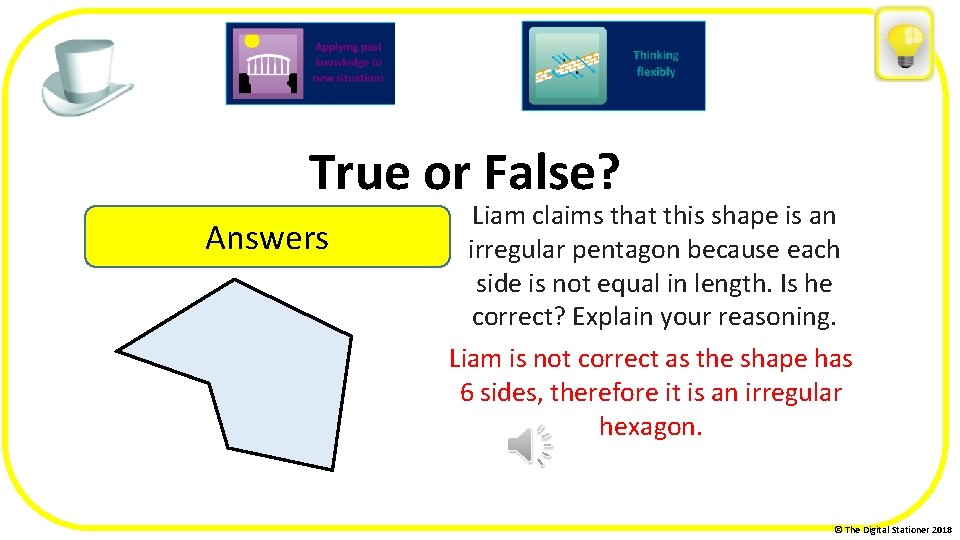True or False? Answers Liam claims that this shape is an irregular pentagon because each side is not equal in length. Is he correct? Explain your reasoning. Liam is not correct as the shape has 6 sides, therefore it is an irregular hexagon. © The Digital Stationer 2018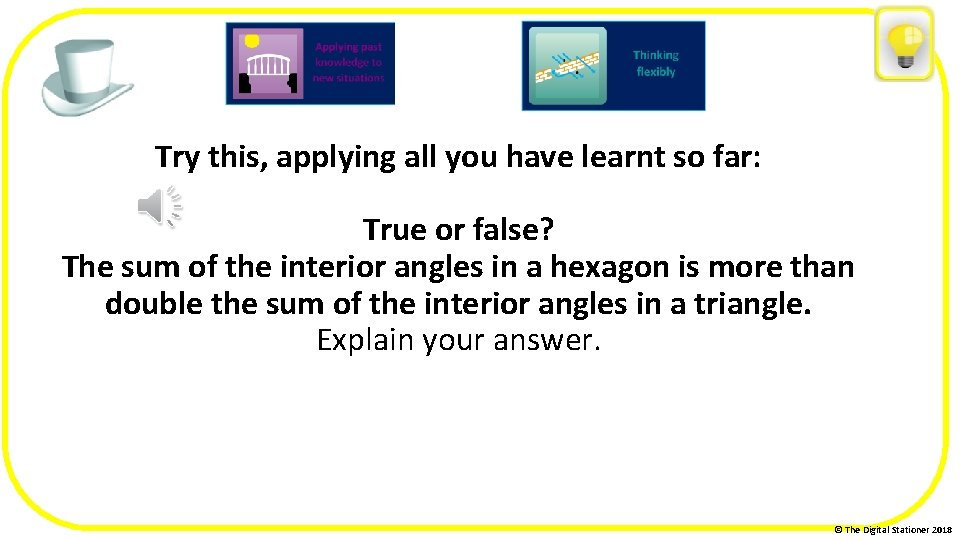Try this, applying all you have learnt so far: True or false? The sum of the interior angles in a hexagon is more than double the sum of the interior angles in a triangle. Explain your answer. © The Digital Stationer 2018Try this, applying all you have learnt so far: True or false? The sum of the interior angles in a hexagon is more than double the sum of the interior angles in a triangle. Explain your answer. A regular hexagon has 6 sides. If we follow the formulae, it is 6 -2 = 4 then 4 x 180 = 720. The sum of the interior angles of a triangle are 180 so this is false. © The Digital Stationer 2018Your Task Today: There are 4 tasks to do today, start on the mildest chilli challenge and work your way through to the super hot challenge.I am an irregular polygon with one right angle. The sum of all angles is 180 o. What irregular polygon am I?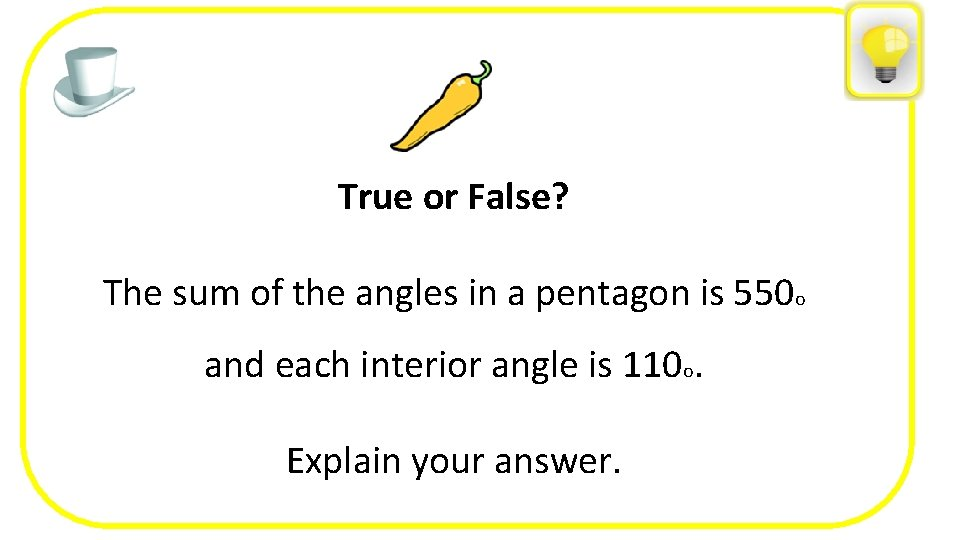True or False? Tara is correct because a turn in either direction will have them facing South. The sum of the angles in a pentagon is 550 o and each interior angle is 110 o. Explain your answer.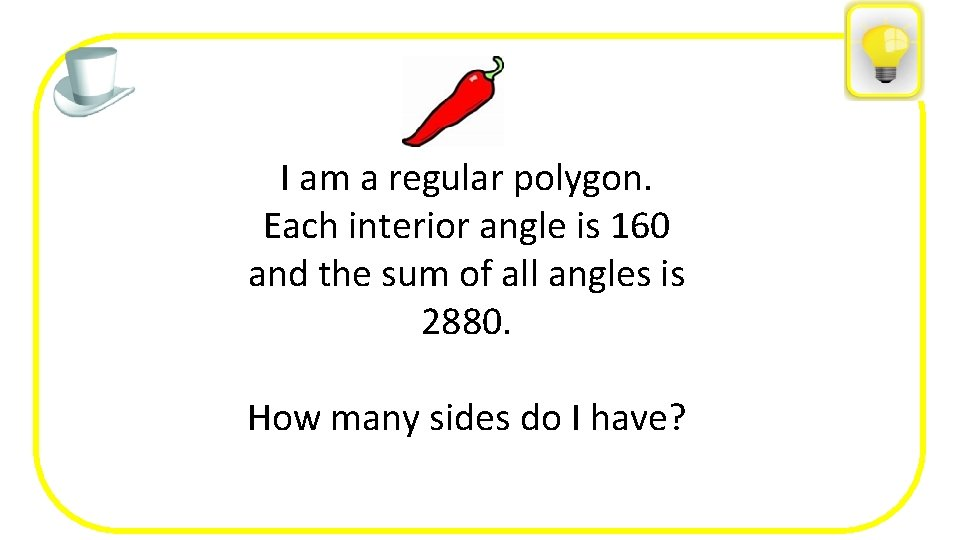I am a regular polygon. Each interior angle is 160 and the sum of all angles is 2880. How many sides do I have?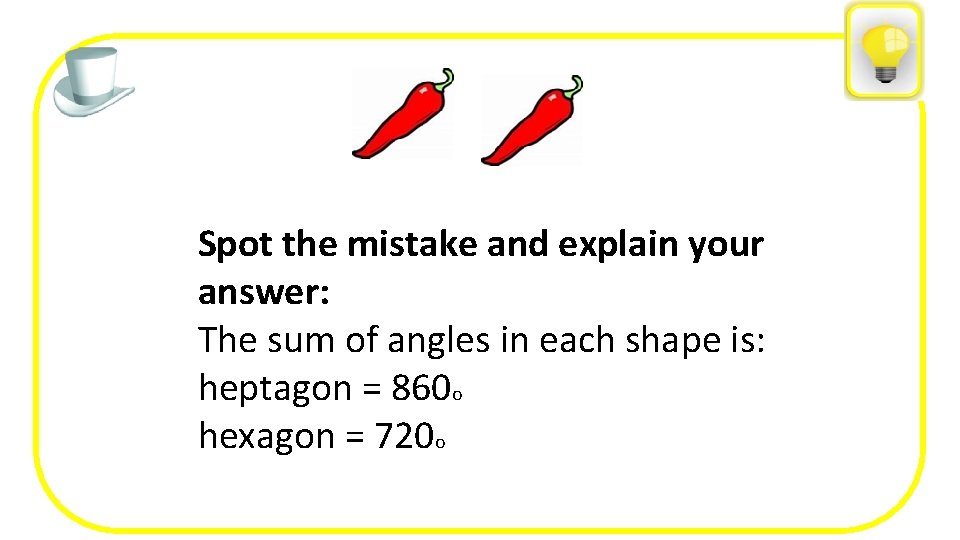Spot the mistake and explain your answer: The sum of angles in each shape is: heptagon = 860 o hexagon = 720 o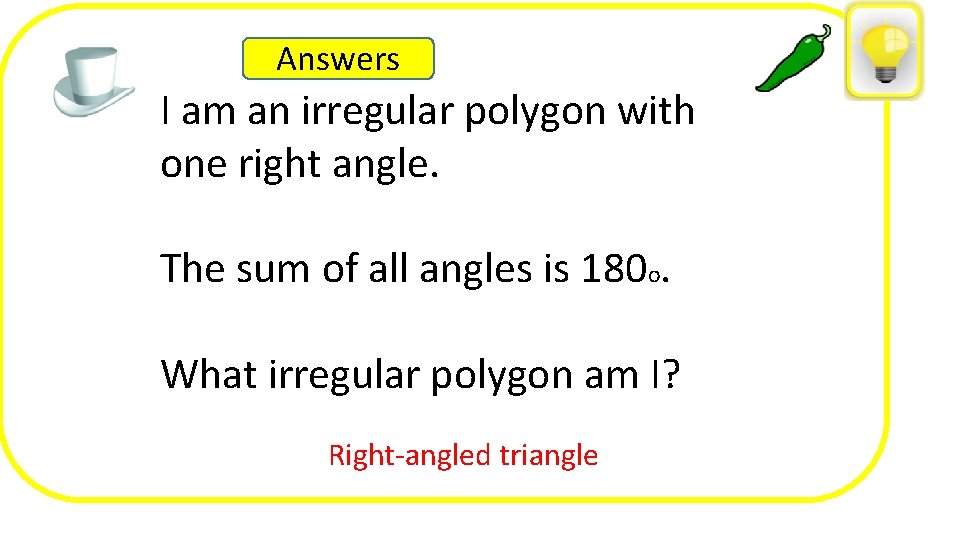Answers I am an irregular polygon with one right angle. The sum of all angles is 180 o. What irregular polygon am I? Right-angled triangle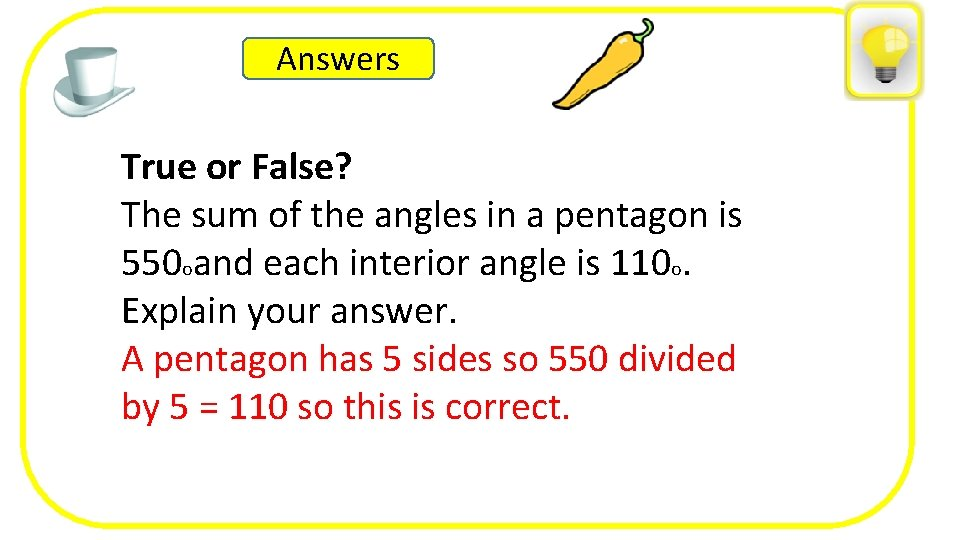Answers True or False? The sum of the angles in a pentagon is Tara is correct a turn in either direction will have them facing South. o. 550 because oand each interior angle is 110 Explain your answer. A pentagon has 5 sides so 550 divided by 5 = 110 so this is correct.Answers I am a regular polygon. Each interior angle is 160 and the sum of all angles is 2880. How many sides do I have? 18 as 2800 o÷ 160 o= 18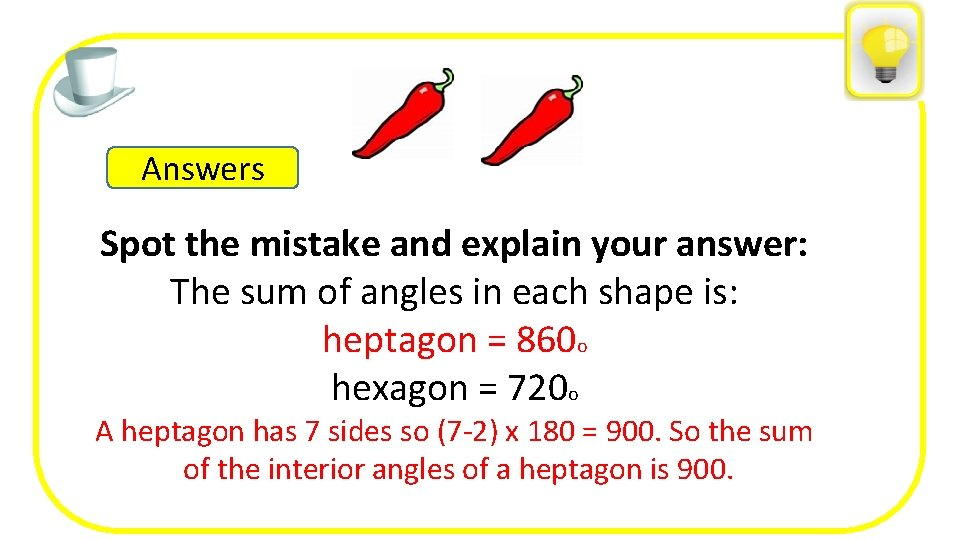Answers Spot the mistake and explain your answer: The sum of angles in each shape is: heptagon = 860 o hexagon = 720 o A heptagon has 7 sides so (7 -2) x 180 = 900. So the sum of the interior angles of a heptagon is 900.